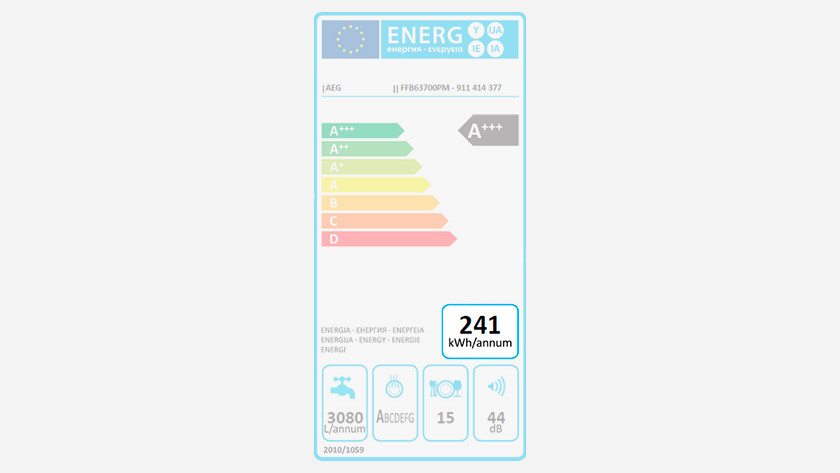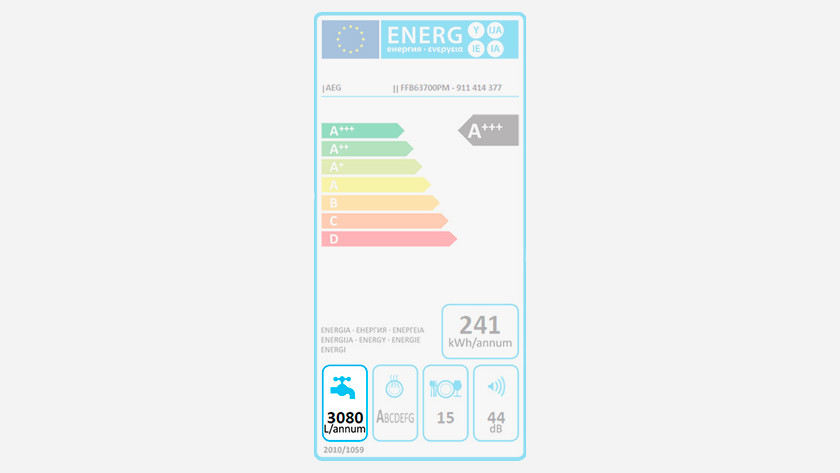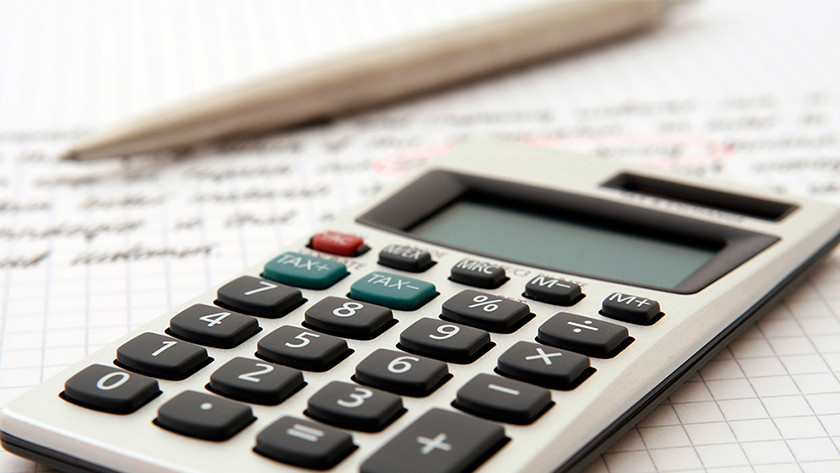Written by Rowan

# How much water and energy does a dishwasher use?

When buying a new dishwasher, of course you want to know if the dishwasher is energy-efficient and makes you save money. The most economical is a dishwasher with energy label A+++. If you choose an A+++-10% model, it's 10% more economical than an A+++ model. In this article, we explain what the energy consumption of a dishwasher is and how high your average annual energy costs are.

## Table

Energy consumption at a time Annual energy consumption Annual energy costs
A+++-10% 0.73 kWh 211kWh € 48.53
A+++ 0.84 kWh 235.86 kWh € 54.25
A++ 0.89 kWh 251.42 kWh € 57.83
A+ 0.90 kWh 255.67 kWh € 58.80

## Energy consumptionOn the energy label of a dishwasher, you can see exactly what the energy label and the consumption is. An A+++ dishwasher is the most economical. If you have an A+++-10% model, it's 10% more efficient. The energy costs from the table in this article are based on 280 cycles per year (more than 5 cycles per week) and an energy price of € 0.23 per kWh.

## Water consumptionTo know the water consumption of a dishwasher, look at the number under the tap on the energy label. You multiply the water consumption in liters per year by € 0.0014. This is the average water price per liter. Example: the energy label states that the water consumption is 3080 liters per year. On average, you'll pay € 4.31 per year for water consumption.

## Formula energy costsDo you want to calculate the average energy costs per yea in eurosr yourself? Do this as follows: € 0.23 x average energy consumption in kWh per year (see energy rating).Article by:
Rowan Dishwasher Expert.

© 1999 - 2020 - Coolblue N.V.
Customer rating: 9.2 / 10 -